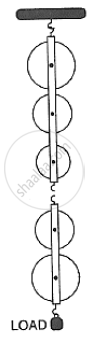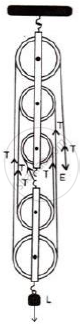Share

Books Shortlist

# In Fig. 3.38, Draw a Tackle to Lift the Load by Applying the Force in the Downward Direction. Mark the Position of Load and Effort. (A) If the Load is Raised by 1 M, Through What Distance Will the Eff - ICSE Class 10 - Physics

ConceptMachines as Force Multipliers

#### Question

In fig. 3.38, draw a tackle to lift the load by applying the force in the downward direction. Mark the position of load and effort.
(a) If the load is raised by 1 m, through what distance will the effort move?
(b) State how many strands of tackle are supporting the load?
(c) What is the mechanical advantage of the system?#### Solution(a) The effort move = 1 × 5 = 5m
(b) Five strands of tackle are supporting the load.
(c) Mechanical advantage of the system = M.A = "𝑙𝑜𝑎𝑑"/"𝑒𝑓𝑓𝑜𝑟𝑡" = "5𝑇"/"𝑇" = 5

Is there an error in this question or solution?

#### APPEARS IN

Solution In Fig. 3.38, Draw a Tackle to Lift the Load by Applying the Force in the Downward Direction. Mark the Position of Load and Effort. (A) If the Load is Raised by 1 M, Through What Distance Will the Eff Concept: Machines as Force Multipliers.
S